Heisenberg Uncertainty Principle

# Heisenberg Uncertainty Principle Notes | Study Physical Chemistry - Chemistry

## Document Description: Heisenberg Uncertainty Principle for Chemistry 2022 is part of Physical Chemistry preparation. The notes and questions for Heisenberg Uncertainty Principle have been prepared according to the Chemistry exam syllabus. Information about Heisenberg Uncertainty Principle covers topics like Introduction – What is Heisenberg’s Uncertainty Principle? and Heisenberg Uncertainty Principle Example, for Chemistry 2022 Exam. Find important definitions, questions, notes, meanings, examples, exercises and tests below for Heisenberg Uncertainty Principle.

Introduction of Heisenberg Uncertainty Principle in English is available as part of our Physical Chemistry for Chemistry & Heisenberg Uncertainty Principle in Hindi for Physical Chemistry course. Download more important topics related with notes, lectures and mock test series for Chemistry Exam by signing up for free. Chemistry: Heisenberg Uncertainty Principle Notes | Study Physical Chemistry - Chemistry
 Table of contents Introduction – What is Heisenberg’s Uncertainty Principle?
 1 Crore+ students have signed up on EduRev. Have you?

Introduction – What is Heisenberg’s Uncertainty Principle?

• Heisenberg’s uncertainty principle states that it is impossible to measure or calculate exactly, both the position and the momentum of an object. This principle is based on the wave-particle duality of matter.
• Although Heisenberg’s uncertainty principle can be ignored in the macroscopic world (the uncertainties in the position and velocity of objects with relatively large masses are negligible), it holds significant value in the quantum world. Since atoms and subatomic particles have very small masses, any increase in the accuracy of their positions will be accompanied by an increase in the uncertainty associated with their velocities.
• In the field of quantum mechanics, Heisenberg’s uncertainty principle is a fundamental theory that explains why it is impossible to measure more than one quantum variables simultaneously. Another implication of the uncertainty principle is that it is impossible to accurately measure the energy of a system in some finite amount of time.

Why is it Impossible to Measure both Position and Momentum Simultaneously?
In order to illustrate Heisenberg’s uncertainty principle, consider an example where the position of an electron is measured. In order to measure the position of an object, a photon must collide with it and return to the measuring device. Since photons hold some finite momentum, a transfer of momenta will occur when the photon collides with the electron. This transfer of momenta will cause the momentum of the electron to increase. Thus, any attempt at measuring the position of a particle will increase the uncertainty in the value of its momentum.
Applying the same example to a macroscopic object (say a basketball), it can be understood that Heisenberg’s uncertainty principle has a negligible impact on measurements in the macroscopic world. While measuring the position of a basketball, there will still be a transfer of momentum from the photons to the ball. However, the mass of the photon is much smaller than the mass of the ball. Therefore, any momentum imparted by the photon to the ball can be neglected.

• Heisenberg uncertainty principle imposes a restriction on the accuracy of simultaneous measurement of position and momentum. The more precise our measurement of position is, the less accurate will be our momentum measurement and vice-versa.
• The physical origin of the Heisenberg uncertainty principle is with the quantum system. Determination of position by performing a measurement on the system disturbs it sufficiently to make the determination of q imprecise and vice-versa. We shall learn about the principle in detail below.
• Heisenberg uncertainty principle states that for particles exhibiting both particle and wave nature, it will not be possible to accurately determine both the position and velocity at the same time.
• The principle is named after  German physicist, Werner Heisenberg who proposed the uncertainty principle in the year 1927. This principle was formulated when Heisenberg was in trying to build an intuitive model of quantum physics.
• He discovered that there were certain fundamental factors that limited our actions in knowing certain quantities.
• This principle basically highlights that simultaneous measurement of position and the velocity or momentum of microscopic matter waves will have an error such that the product of the error in measurement of position and momentum is equal or more than an integral multiple of a constant.

Heisenberg Uncertainty Principle Formula and Application

If, Δx is the error in position measurement and Δp is the error in the measurement of momentum, then
Δx × Δp ≥ h/4π
Since momentum, p = mv, Heisenberg’s uncertainty principle formula can be alternatively written as-
Δx × Δmv ≥ h/4π or ΔX  ×  Δm × Δv  ≥ h/4π
Where, ΔV is the error in the measurement of velocity and assuming mass remaining constant during the experiment,
ΔX  ×  ΔV  ≥ h/4πm
Accurate measurement of position or momentum automatically indicates larger uncertainty (error) in the measurement of the other quantity.
Applying the Heisenberg principle to an electron in an orbit of an atom, with h = 6.626 ×10-34Js and m= 9.11 ×10-31Kg,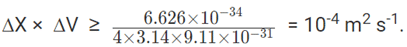If the position of the electron is measured accurately to its size (10-10m), then the error in the measurement of its velocity will be equal or larger than 106m or 1000Km.
Heisenberg principle applies to only dual-natured microscopic particles and not to a macroscopic particle whose wave nature is very small.

Explaining Heisenberg Uncertainty Principle With An Example

• Electromagnetic radiations and microscopic matter waves exhibit a dual nature of mass/ momentum and wave character.
• Position and velocity/momentum of macroscopic matter waves can be determined accurately, simultaneously. For example, the location and speed of a moving car can be determined at the same time, with minimum error. But, in microscopic particles, it will not be possible to fix the position and measure the velocity/momentum of the particle simultaneously.
• An electron in an atom has a mass of 9.91 × 10-31Kg. Naked eyes will not see such small particles. A powerful light may collide with the electron and illuminate it. Illumination helps in identifying and measuring the position of the electron.
• The collision of the powerful light source, while helping in identification increases the momentum of the electron and makes it move away from the initial position. Thus, when fixing the position, velocity /momentum of the particle would have changed from the original value.
• Hence, when the position is exact, error occurs in the measurement of velocity or momentum. In the same way, the measurement of momentum accurately will change the position.
• Hence, at any point in time, either position or momentum can only be measured accurately.
• Simultaneous measurement of both of them will have an error in both position and momentum. Heisenberg quantified the error in the measurement of both position and momentum at the same time.

Heisenberg’s γ-ray Microscope

A striking thought experiment illustrating the uncertainty principle is Bohr’s / Heisenberg’s Gamma-ray microscope. To observe a particle, say an electron, we shine it with the light ray of wavelength λ and collect the Compton scattered light in a microscope objective whose diameter subtends an angle θ with the electron as shown in the figure below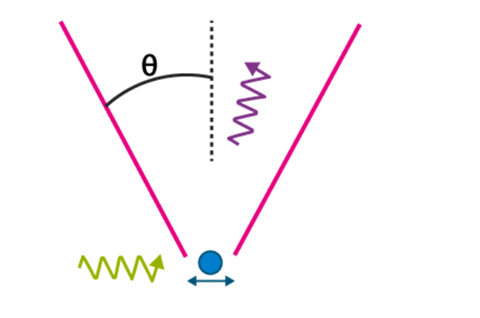The precision with which the electron can be located, Delta x, is defined by the resolving power of the microscope,
sinθ = λ/Δx ⇒ Δx = λ/sinθ
It appears that by making λ small, that is why we choose γ-ray, and by making sin θ large, Delta x can be made as small as desired. But, according to the uncertainty principle, we can do so only at the expense of our knowledge of x-component of electron momentum.
In order to record the Compton scattered photon by the microscope, the photon must stay in the cone of angle θ and hence its x-component of the momentum can vary within ±(h/λ) sin θ. This implies, the magnitude of the recoil momentum of the electron is uncertain by
Δpx = 2h/λ sinθ
The product of the uncertainty yields,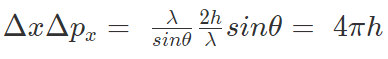Is Heisenberg’s Uncertainty Principle Noticeable in All Matter Waves?

Heisenberg’s principle is applicable to all matter waves. The measurement error of any two conjugate properties, whose dimensions happen to be joule sec, like position-momentum, time-energy will be guided by the Heisenberg’s value.
But, it will be noticeable and of significance only for small particles like an electron with very low mass. A bigger particle with heavy mass will show the error to be very small and negligible.

Heisenberg Uncertainty Principle Equations

Heisenberg’s uncertainty principle can be considered as a very precise mathematical statement that describes the nature of quantum systems. As such, we often consider two common equations related to the uncertainty principle. They are;
Equation 1: ΔX ⋅ ∆p ~ ħ
Equation 2:  ∆E ⋅ ∆t ~ ħ
Where,
ħ = value of the Planck’s constant divided by 2*pi
ΔX = uncertainty in the position
∆p = uncertainty in momentum
∆E = uncertainty in the energy
∆t = uncertainty in time measurement

Solved Numerical Problems on Heisenberg’s Uncertainty Principle

1. If the position of the electron is measured within an accuracy of + 0.002 nm, calculate the uncertainty in the momentum of the electron. Suppose the momentum of the electron is h / 4pm × 0.05 nm, is there any problem in defining this value.
Solution:
a) ∆x = 2×10-12m; ∆X  ×  ∆mV  ≥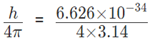∵ ∆mV ≥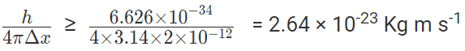b) Momentum mv =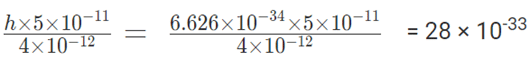Error in momentum measurement is 1010 times larger than the actual momentum. The given momentum will not be acceptable.

2. Position of a chloride ion on a material can be determined to a maximum error of 1μm. If the mass of the chloride ion is 5.86 × 10-26Kg, what will be the error in its velocity measurement?
Solution:
∆x =  10-6 m; ∆X  ×  ∆mV  ≥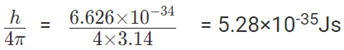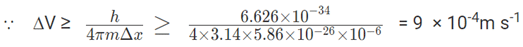3. The lifetime of an excited state of an atom is 3 × 10-3s. What is the minimum uncertainty in its energy in eV?
Solution: Time and energy are conjugate pairs with Js unit. The product of measurement error is given by Heisenberg’s principle.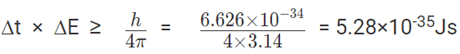Assuming a maximum error in the measurement of lifetime equal to that of lifetime = 3 ×10-3s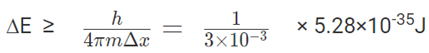⸪ 1 Joule = 6.242 × 1018ev,
Uncertainty in the determination of energy of the atom = ∆E = 6.22 × 1018 × 1/3×10-3 × 5.28 ×10-35
= 1.1×10-13

4. A wet ball weighing 10.1gm has a water of 0.1g on it. The ball is moving with a constant velocity with an uncertainty of momentum of 10-6 kg m/s. What will be the uncertainty in the measurement of the position of the ball, water and electron in the water molecule?
Solution: ∆X × ∆p ≥ h/4π
Velocity being constant, uncertainty in the measurement of the momentum is associated with the mass of the matter.
Uncertainty in the momentum of the dry ball = mass ×10-6   = 10×10-3×10-6 Kg m s-1.
Uncertainty in the momentum of the water    = mass ×10-6 = 0.1×10-3×10-6  Kg m s-1.
Uncertainty in the momentum of the electron = mass ×10-6 = 9×10-31×10-6  Kg m s-1.
Uncertainty in position measurement is inversely proportional to the uncertainty in momentum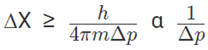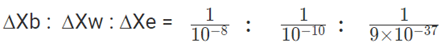=    108  :    1010   :    1.1 × 1036   or
=  1      :   102     :    1028

4. Determine the minimum uncertainties in the positions of the electron if their speeds are known with a precision of 3.0×10−3m/s?
Solution:
Δu=3.0×10−3m/s
Uncertained momentum Δp=m Δu
Uncertainty in position Δx=/(2Δp)
For electron
Δp=m Δu
= (9.1×10−31kg × 3.0×10−3)
Δp  = 2.73 × 10-33 kg.m/s
Δx = h/Δp
Δx = 0.12 m

The document Heisenberg Uncertainty Principle Notes | Study Physical Chemistry - Chemistry is a part of the Chemistry Course Physical Chemistry.
All you need of Chemistry at this link: Chemistry

## Physical Chemistry

67 videos|106 docs|31 tests
 Use Code STAYHOME200 and get INR 200 additional OFF

## Physical Chemistry

67 videos|106 docs|31 tests

Track your progress, build streaks, highlight & save important lessons and more!

,

,

,

,

,

,

,

,

,

,

,

,

,

,

,

,

,

,

,

,

,

;